# MCQs on Control Systems

##### Page 10 of 23. Go to page 1 2 3 4 5 6 7 8 9 10 11 12 13 14 15 16 17 18 19 20 21 22 23
01․ The DC gain of a system represented by the transfer function 12 / {( s + 2 )( s + 3 )} is
1.
2.
5.
10.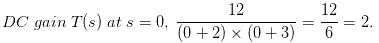02․ The transfer function of a system given by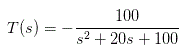The system is
an over damped.
a critically damped.
an under damped.
unstable.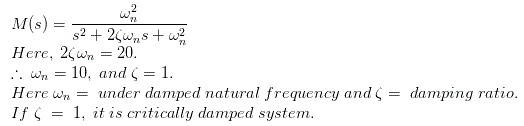03․ Find the order of a system given by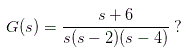2.
3.
4.
5.

Generally order of the system is given by the highest power of “s” in denominator of transfer function. Here, the highest power of S is 3 in the denominator of transfer function.

04․ Find the type of the system given by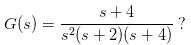2.
3.
4.
1.

Type of the system is determined from the no. of poles located at the origin. Here the no. of poles at origin = 2.

05․ A negative feedback closed loop control system is supplied to an input of 5 volt. The system has a forward gain of 1 and a feedback gain of 1. What is the output voltage?
1.0 Volt.
1.5 Volt.
2.5 Volt.
2.0 Volt.

We know that negative feedback closed loop system ,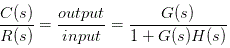Where G ( s ) = Forward gain, H ( s ) = Feedback gain.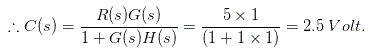06․ A system of constant voltage and constant frequency is called _________________ system.
feedback.
infinite.
zero.
none of the above.

A system of constant voltage and constant frequency regardless of load is called infinite bus bar system.

07․ None of the poles of a linear control system lie in the right half of s plane. For a bounded input, the output of this system
always bounded.
could be unbounded.
tends to zero.
none of these.

For a linear control system with no poles in RHS of s plane including roots on j axis with bounded input, the output may be unbounded.

08․ Number of sign changes in the entries in 1st column of Routh array denotes the no. of
roots of characteristic polynomial in RHP.
zeroes of system in RHP.
open loop poles in RHP.
open loop zeroes in RHP.

Number of sign changes in the 1st column of Routh array denotes number of roots of the characteristic equation in RHS of s plane.

09․ A cascade of three linear time invariant systems is causal and unstable. From this we conclude that
each system in the cascade is individually caused and unstable.
at least one system is unstable and at least one system is causal.
at least one system is causal and all systems are unstable.
the majority are unstable and the majority are causal.

There must be at least one system causal and one unstable out of three for the whole system to be causal and unstable.

10․ In the integral control of the single area system frequency error is reduced to zero. Then
integrator output and speed changer position attain a constant value.
integrator o/p decreases but speed changer position moves up.
integrator o/p increases but speed changer position comes down.
integrator o/p decreases and speed changer position comes down.

In the integral control of single area system, when the system frequency error is reduced to zero, the integrator output and the speed changer position attain a constant value.

<<<89101112>>>